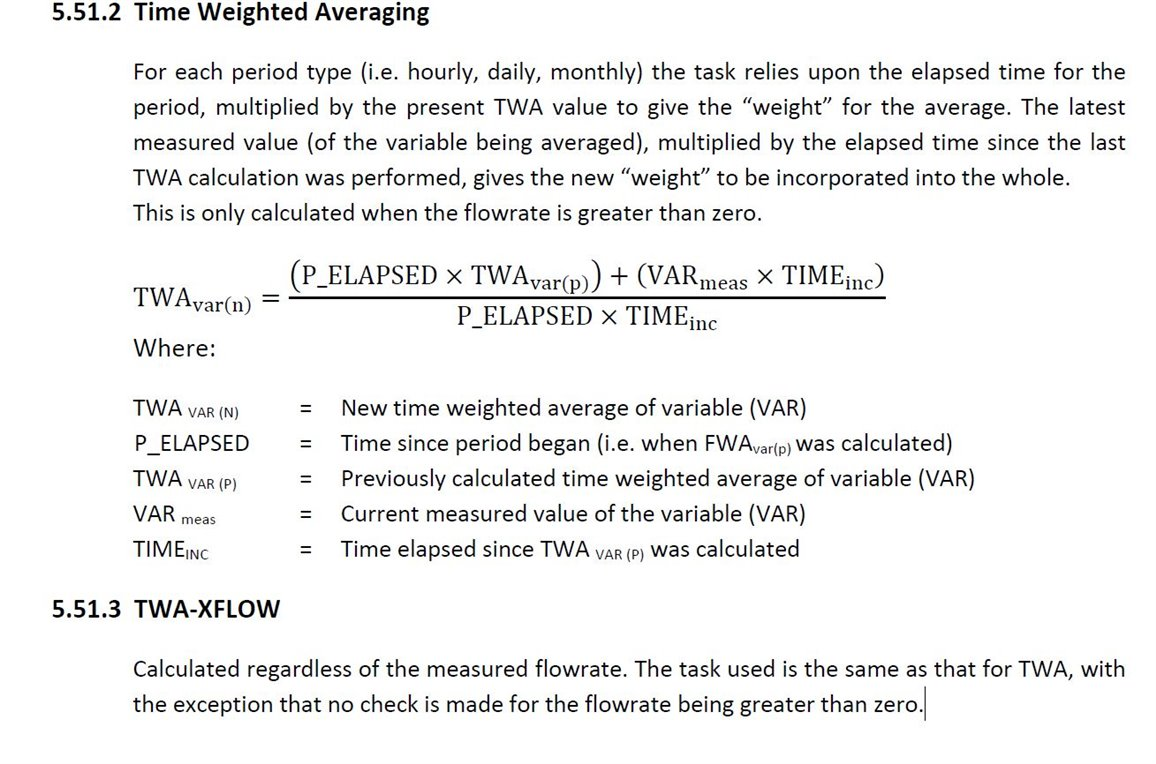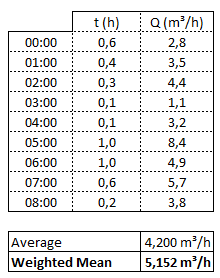### Time and Flow Weighted Average at the S600 Floboss

Dear fellows,

I'm trying to understand the definition mentioned for the TWA method used by the S600 to calculate the flow average but couldn't get much further.

I fount the definition at the manual extremely confusing as shown below:

The only thing I need to assure is that the TWA takes into account JUST the periods where the flow isn't zero an then returns me the flow weighted mean using the time as "weight" except when there is no flow. Is this the calculation that the TWA actually does?

For each period type (i.e. hourly, daily, monthly) the task relies upon the elapsed time for the period, multiplied by the present TWA value to give the “weight” for the average. The latest measured value (of the variable being averaged), multiplied by the elapsed time since the last TWA calculation was performed, gives the new “weight” to be incorporated into the whole. This is only calculated when the flow rate is greater than zero.

Kind regards,

Vitor

•T

• In reply to ian firth:

Thank you Ian but I have a lot of trouble to understand this formula. If my understandings are correct, the TWA is the weighted average of the flow taking in account ONLY the time periods when the flow is greater than zero, is that it?
I'm confused because the formula states that it's weighting the AVERAGE OF THE VARIABLE with time instead of the FLOW with time, if you are averaging the average with time the final result would be something else then the flow weighted mean, right?

• We have
Flow Weighted (FWA) which will average the PV based on the total accumulated (i.e. based on the flow)
Time Weighted (TWA) which will average the PV based on time – but will only calculate the average if there is flow (but it doesn’t use the flow in the calculation).
Time Weighted without Flow (TWA-XFLOW) which will average the PV based on time – but will only calculate the average if there is no flow .

as we have more info available for you if you have done the advanced training courses etc as well as latest software and binary files
• In reply to ian firth:

Ian, once more thanks for the reply.

Just wanna make sure I got things straight, the TWA then would return me the bolded value and the FWA would retrieve the not-bolded value. Is that it?

Also, I still don't get it when you say that it "doesn't use the flow in the calculation". I can't think of any other way to get the weighted mean than multiplying the flow by the time "it flowed" and dividing everything by the total flow time.Your calculations look correct. I have attached workings and also the FWA part of the text I missed on my initial response

• In reply to ian firth:

Ian, once more thanks again for your help and concern.

Finally, the FWA actually returns the flow weighted average discarding the time periods on which the flow is zero, right?

I still have trouble to figure out how that formula works, tough.

For example, on the numeric example a proposed, which numeric values from the table would correspond to P_TOTAL , FWAVAR(P), VARmeas and TOTALinc ?